## 第三次作业--24点游戏_c++ judgement函数_A.y.的博客-程序员秘密

24点游戏是经典的纸牌益智游戏。

1.程序风格良好(使用自定义注释模板)
2.列出表达式无重复。

1. 程序风格良好(使用自定义注释模板)
2.使用计时器要求用户在规定时间内输入表达式，如果规定时间内运算正确则加分，超时或运算错误则进入下一题并减少生命值（不扣分）。
3.所有成绩均可记录在TopList.txt文件中。

1.基本要求:

caculate()函数返回两数进行基本操作后的值；
judgement()函数里面先将4个随机数的任意两个

2.提高要求

1则进行’+’ ‘-’操作,若symbol==2则进行’’ ‘/’操作;
Init()函数初始化栈;
Push()函数将操作数入栈;
Pop()函数将栈顶元素取出;
dispaly()函数将表达式键入并用getchar()函数对输入的每个字符进行判断,若为个位整数,则入栈,若为’+’ ‘-’符则栈顶元素symbol为1,若为’
’ ‘/’符则栈顶元素symbol为2,若为’(‘符则lay为1,若为’)’符则lay为0；判断栈顶第二个元素symbol的值,若为0,为出栈两个操作数合成十位数再入栈,若为2且lay不为1则出栈两个数进行’*’或‘/’操作后将结果入栈,若为1且lay为1则进行’+’ ‘-’操作后将结果入栈;上述操作完后出栈栈顶元素,返回栈顶元素。

game()函数将display()输入的表达式运算结果进行判断,若为24且键入表达式时间(end-start)不多于30s,则得分+1,否则生命值-1,若生命值为0则游戏结束。

1.基本要求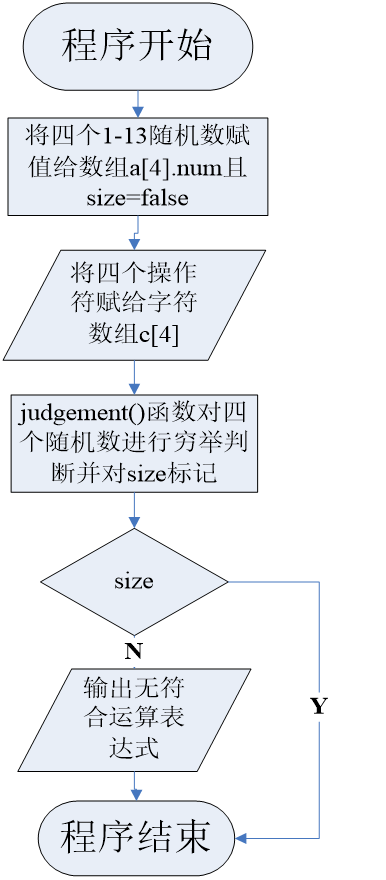2.提高要求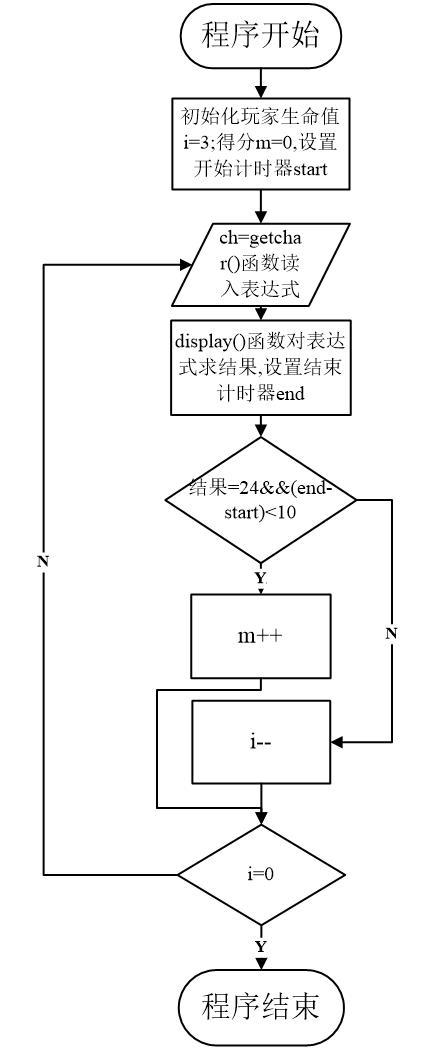``````#include<stdio.h>
#include<stdlib.h>
#include<time.h>
#include<string.h>
#define MAX 20
bool size=false;                                 /*标志是否有运算结果,是则为true,否则为false*/
int line=1;
typedef struct                                   /*定义操作数结构体,a为操作数的值,symbol标示进行的操作*/
{

double a;
int symbol;

typedef struct
{

int top;
}seqlist;

seqlist *Init()                                /*初始化栈*/
{

seqlist *s;
s=(seqlist *)malloc(sizeof(seqlist));
s->top=-1;
return s;
}

int Push(seqlist *s,double x)                    /*将操作数入栈*/
{

s->top++;
s->data[s->top].a=x;
s->data[s->top].symbol=0;
return 1;
}

int Pop(seqlist *s,double *x)
{

*x=s->data[s->top].a;
s->top--;
return 1;
}
struct Number                               /*24点随机四个数*/
{

int symbol;
double num;
}a;

double caculate(double num1,double num2,char caculate) /*计算两数的操作结果*/
{

switch(caculate)
{

case '+':
return(num1+num2);
case '-':
return(num1-num2);
case '*':
return(num1*num2);
case '/':
return(num1/num2);
}
return 0;
}

void judgement(char c)                        /*列出四种数所有可能的情况*/
{

int i,j,m,n,o,p,q;
double temp1,temp2,temp3;
for(i=0;i<4;i++)
{

for(j=i+1;j<4;j++)
{

for(o=0;o<4;o++)                     /*四种数任取两种先进行运算*/
{

temp1=double(caculate(a[i].num,a[j].num,c[o]));
a[i].symbol=0;
a[j].symbol=0;
for(m=0;m<4;m++)
{

if(a[m].symbol!=0)
{

for(p=0;p<4;p++)         /*任取剩余一个数与上一个结果进行运算*/
{

temp2=caculate(temp1,a[m].num,c[p]);
a[m].symbol=0;
for(n=0;n<4;n++)
{

if(a[n].symbol!=0)
{

for(q=0;q<4;q++)  /*将最后一个数与上一次结果进行运算得到结果*/
{

temp3=caculate(temp2,a[n].num,c[q]);
if(temp3==24) /*若结果等于24,则打印出该表达式*/
{

if(o<2&&p<2&&q<2)
printf("\n%d%c%d%c%d%c%d",int(a[i].num),c[o],int(a[j].num),c[p],int(a[m].num),c[q],int(a[n].num));
else if(o<2&&p<2&&q>=2)
printf("\n(%d%c%d%c%d)%c%d",int(a[i].num),c[o],int(a[j].num),c[p],int(a[m].num),c[q],int(a[n].num));
else if(o<2&&p>=2)
printf("\n(%d%c%d)%c%d%c%d",int(a[i].num),c[o],int(a[j].num),c[p],int(a[m].num),c[q],int(a[n].num));
else if(o>2&&p<2&&q>2)
printf("\n((%d%c%d)%c%d)%c%d",int(a[i].num),c[o],int(a[j].num),c[p],int(a[m].num),c[q],int(a[n].num));
else if(o>2)
printf("\n%d%c%d%c%d%c%d",int(a[i].num),c[o],int(a[j].num),c[p],int(a[m].num),c[q],int(a[n].num));
size=true;
}
}
}
}
a[m].symbol=1;
}
}
}
a[j].symbol=1;
}
}
a[i].symbol=1;
}
}

int display()                                    /*对输入的表达式进行运算并判断的函数*/
{

seqlist *s;
char ch,ch2;
char ch3[MAX];
int i=0,lay;
double o1,o2,temp,ch1;                       /*o1表示栈顶元素,o2表示栈顶第二个元素,temp临时存储两数操作后的值*/
s=Init();
printf("\n\n\t\t请输入表达式:");
while((ch=getchar())!='\n')
{

if(ch==' ')
continue;
if(ch>47&&ch<58)
{

ch1=ch-48;
Push(s,ch1);
}
else if(ch=='+'||ch=='-')
{

s->data[s->top].symbol=1;
ch3[i++]=ch;
continue;
}
else if(ch=='*'||ch=='/')
{

s->data[s->top].symbol=2;
ch2=ch;
continue;
}
else if(ch=='(')
{

lay=1;
}
else if(ch==')')
{

lay=0;
}
if(lay!=1&&s->data[s->top-1].symbol==2)
{

Pop(s,&o1);
Pop(s,&o2);
temp=caculate(o2,o1,ch2);
Push(s,temp);
}
if(lay==1&&s->data[s->top-1].symbol==1)
{

Pop(s,&o1);
Pop(s,&o2);
temp=caculate(o2,o1,ch3[--i]);
Push(s,temp);
}
if(s->top!=0&&s->data[s->top-1].symbol==0)
{

Pop(s,&o1);
Pop(s,&o2);
temp=o2*10+o1;
Push(s,temp);
}
}
for(int j=i;j>0;j--)
{

Pop(s,&o1);
Pop(s,&o2);
temp=caculate(o2,o1,ch3[j-1]);
Push(s,temp);
}

Pop(s,&o1);
return((int)o1);
}

void game()                                      /*开始游戏*/
{

FILE *fp;
fp=fopen("TopList.txt","at");
int i,j,m,sum;
double temp;
clock_t end,start;                           /*设置计时器计算键入表达式时间*/
i=3;
m=0;
printf("             *************输入表达式请在30s内输入*************\n\n\n");
while(i)
{

if(line!=0)
{

line--;
if(getchar()=='\n')
continue;
}
srand((int)time(0));
for(j=0;j<4;j++)
{

sum[j]=rand()%13+1;
}
printf("\t四个随机数为:");
for(j=0;j<4;j++)
printf("%-4d",(int)sum[j]);
start=clock();                           /*记录刚开始输入表达式时间*/
if(display()==24)
{

end=clock();                         /*记录输入表达式后时间*/
temp=(double)(end-start)/1000;
if(temp<30)
m++;
else
{

printf("\t\t输入超时!");
i--;
}
}
else
i--;
printf("\n\t\t\t\t得分:%d\n\n",m);
}
fprintf(fp,"该次游戏的总得分为:%d\n\n",m);
fclose(fp);
printf("***----------------游戏结束----------------***\n");
}

{

int choose;
printf("\n\t\t请选择是否进行游戏:");
printf("\n\t\t\t1.进行游戏");
printf("\n\t\t\t2.结果程序");
printf("\n\n\t\t选择序号:");
scanf("%d",&choose);
switch(choose)
{

case 1:
system("cls");
game();
break;
case 2:
return;
}
}

int main()
{

char c={
'+','-','*','/'};
srand((int)time(0));
for(int i=0;i<4;i++)
{

a[i].num=rand()%13+1;
a[i].symbol=1;
}
printf("四个随机数为:");
for(i=0;i<4;i++)
printf("%4d",(int)a[i].num);
printf("\n可满足表达式:");
judgement(c);
if(!size)
printf("无!");
printf("\n");
return 0;
}
``````

1.judgement()函数无法输出小括号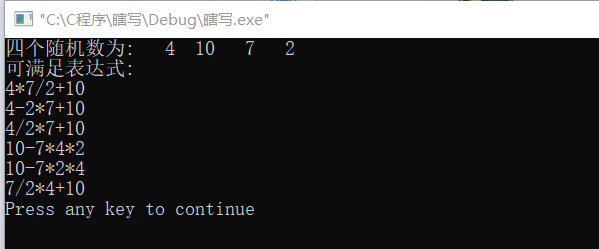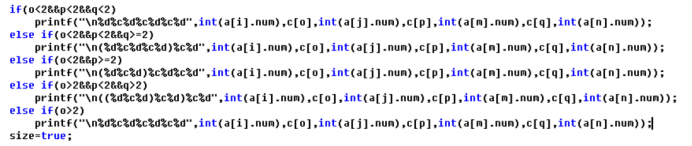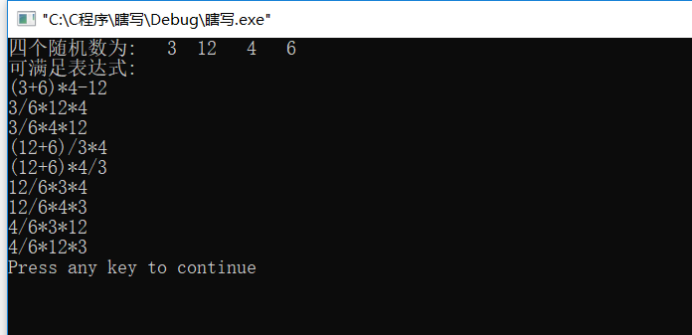display()函数: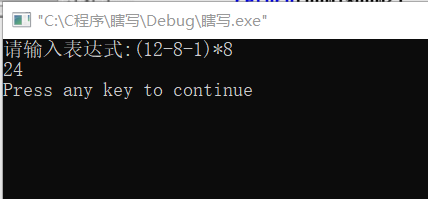judgement()函数: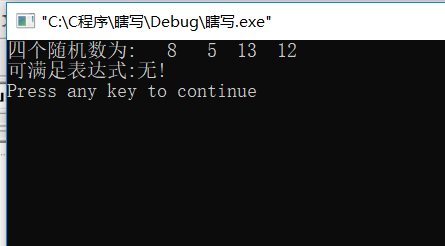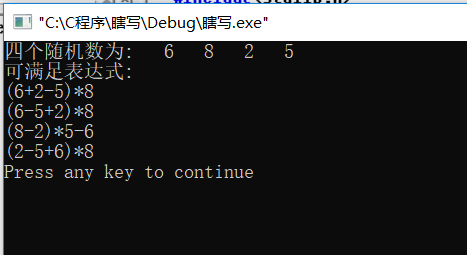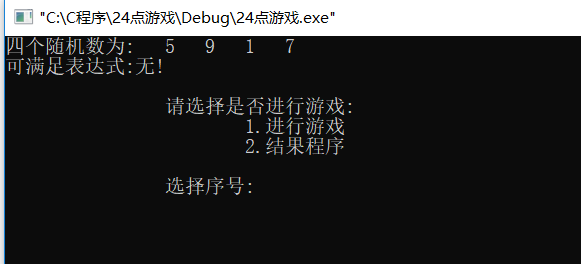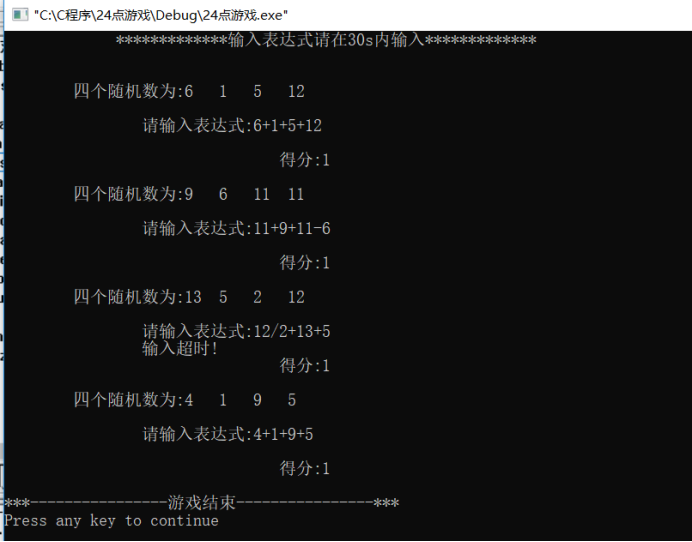### DATEPART() 函数_datepart函数_月亮哥的博客-程序员秘密

SQL Server DATEPART() 函数 定义和用法DATEPART() 函数用于返回日期/时间的单独部分，比如年、月、日、小时、分钟等等。语法DATEPART(datepart,date)date 参数是合法的日期表达式。datepart 参数可以是下列的值： datepart缩写年yy, yyyy季度qq,

### Spring Boot 之FilterRegistrationBean --支持web Filter 排序的使用_你走开``的博客-程序员秘密

Spring Boot 之FilterRegistrationBean  –支持web Filter 排序的使用Spring 提供了FilterRegistrationBean类，此类提供setOrder方法，可以为filter设置排序值，让spring在注册web filter之前排序后再依次注册。  写一个普通的filter：[java] view plain copy print?pac

### Linux服务器集群系统(三)_linux服务器集群分类_p312011150的博客-程序员秘密

LVS集群中的IP负载均衡技术章文嵩 ([email protected]) 2002 年 4 月本文在分析服务器集群实现虚拟网络服务的相关技术上，详细描述了LVS集群中实现的三种IP负载均衡技术（VS/NAT、VS/TUN和VS/DR）的工作原理，以及它们的优缺点。1.前言在 前面文章中，讲述了可伸缩网络服务的几种结构，它们都需要一个前端的负载调度器（或者多个进行主从备份）...

### VGG网络：网络改造的首选基础网络_vgg改进_essenge的博客-程序员秘密

import torch.nn as nnimport torchclass VGG(nn.Module): def __init__(self, features, num_classes=1000, init_weights=False): super(VGG, self).__init__() self.features = features self.classifier = nn.Sequential( nn.D

### Linux【实操篇】—— Shell函数、Shell编程综合案例(定时备份数据库)_shell编程综合设计_敬 之的博客-程序员秘密

Linux 操作系统Shell编程快速入门、shell函数，Shell编程综合案例（定时备份数据库）。

### ABAP (转)BDC常见问题_abap bdc_yiyuzz的博客-程序员秘密

BDC在FICO模块是经常用到的，用于批量过账程序时使用的，但是稳定性不如使用BAPI的好，但是BDC可以有多种T-CODE进行过账，也有不同的模式，功能也不错，但是在写程序或者是出现问题的时候需要考虑下面几个问题：1、可以在程序加入BDC的模式选择，默认为N模式，但是可以选择A模式，方便调试。当然这个也可以debug进去之后进行改值。2、LOOP里面有call BDC的情况，在每一次ca...

### springBoot+dubbo+nacos_code_agent的博客-程序员秘密

1.引入 &lt;properties&gt; &lt;dubbo-spring-boot-starter.version&gt;2.7.5&lt;/dubbo-spring-boot-starter.version&gt; &lt;dubbo-registry-nacos.version&gt;2.7.5&lt;/dubbo-registry-nacos.version&gt; &lt;/properties&gt; &lt;dependencies&g

### pytorch常用函数之torch.randn()_torch.randn函数_土豆豆豆豆豆的博客-程序员秘密

torch.randn(*sizes, out=None)→ Tensor功能：从标准正态分布（均值为0，方差为1）中抽取的一组随机数。返回一个张量sizes (int…) - 整数序列，定义输出张量的形状out (Tensor, optinal) - 结果张量eg：random = torch.randn(2, 3）out: 0.5419 0.1594 -0.0413...